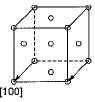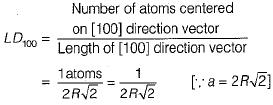# Structural Of Metals & Alloys - 2

## 10 Questions MCQ Test Topicwise Question Bank for GATE Mechanical Engineering | Structural Of Metals & Alloys - 2

Description
This mock test of Structural Of Metals & Alloys - 2 for Mechanical Engineering helps you for every Mechanical Engineering entrance exam. This contains 10 Multiple Choice Questions for Mechanical Engineering Structural Of Metals & Alloys - 2 (mcq) to study with solutions a complete question bank. The solved questions answers in this Structural Of Metals & Alloys - 2 quiz give you a good mix of easy questions and tough questions. Mechanical Engineering students definitely take this Structural Of Metals & Alloys - 2 exercise for a better result in the exam. You can find other Structural Of Metals & Alloys - 2 extra questions, long questions & short questions for Mechanical Engineering on EduRev as well by searching above.
QUESTION: 1

### Surface imperfections that separate two orientations that are mirror image of one- another is called

Solution:

The grain boundary defects are called twin boundary when the surface imperfect orientations on the side are mirror image of those in the opposite side.

QUESTION: 2

### What is the linear density for FCC  direction in terms of the atomic radius?

Solution:QUESTION: 3

### Which of the following regions of the electromagnetic spectrum would be used to determine the structure of crystalline solids?

Solution:

X-ray crystallography: A technique in which the patterns formed by the diffraction of X-rays on passing through a crystalline substance yield information on the lattice structure of the crystal, and the molecular structure of the substance.

QUESTION: 4

The defect responsible for the phenomena of slip, by which most metals deform plastically, is known as

Solution:
QUESTION: 5

The correct order of co-ordination number in SC, BCC, FCC and HCP unit cells is

Solution:
QUESTION: 6

The maximum radius of the interstitial sphere that can fit into the void between the body centred atom of BCC structure is

Solution:
QUESTION: 7

Magnesium crystallizes in HCP structure. If the lattice constant is 0.32 nm, the nearest neighbour distance in magnesium is

Solution:
QUESTION: 8

The packing factor of diamond cubic crystal structure is

Solution:
QUESTION: 9

If (3 2 6) are the Miller indices of a plane, the intercepts made by the plane on the three crystallographic axes are

Solution:
QUESTION: 10

In a simple cubic lattice d100: d110: d111 is
where d is interpianer spacing.

Solution:

Explanation : d100 = a/(12 + 02 + 02)1/2

= a

d110 = a/(12 + 12+ 02)1/2

= a/(2)1/2

d111 = a/(12 + 12 + 12)1/2

= a/(3)1/2

d100 : d110 : d111 = a : a/(2)1/2 : a/(3)1/2

= 61/2 : 31/2 : 21/2